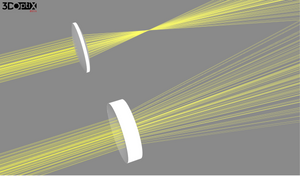August 26, 2020

# Optical Lens - types and main differences

A lens is a transparent material (glass or plastic) with at least one curved surface. The word “lens” derives from the Latin word for "lentil" bean which has the shape of a Bi-convex lens.

The lens shape causes light rays to bend in a specific way as they pass through the lens itself, whether converging to a specific point or diverging as if from a specific point.

• A convex (converging) lens - converges parallel rays of light at a single point on the optical axis, on the opposite side of the lens.
• A concave (diverging) lens - diverge parallel rays. All rays that enter the lens, parallel to its optical axis diverge.

Index of refraction and Snell’s law - The difference between the index of refraction of the lens and the surrounding air causes the actual bending of the light rays. The bending phenomenon is described by Snell’s law for refraction. The law describes the relationship between the angle of incidence and refraction when passing a boundary between two different materials. It relates to the difference in the angle between the incident and refracted light rays to the indices of refraction for two materials.

• Converging lens, refraction rule - Parallel rays traveling to the principal axis of a converging lens (biconvex, plano-convex) refracts through the lens and travel through the focal point on the opposite side of the lens.
• Diverging lens, refraction rule - Parallel rays traveling to the principal axis of a diverging lens (biconcave, plano-concave) refracts through the lens and travel in line with the focal point

Four main spherical lens type

Biconvex lens is the best option when the object and the image are at an equal (or near-equal) distance from the lens which creates a magnification ratio of 1:1. The symmetry reduces spherical aberration as well as canceled chromatic aberration. The same radius of curvature on both spherical surfaces reduces the spherical aberration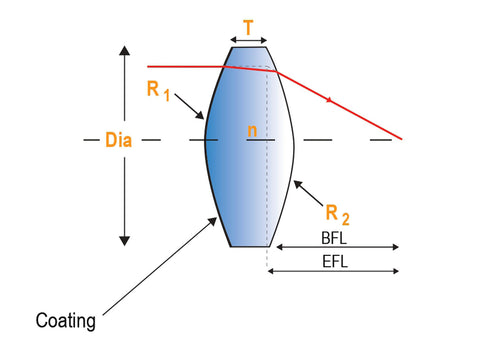Converging or positive lens Curvature on both sides of the lens Thicker at its center than at its edges The focal length is defined as positive Two focal points  Forming a real or a virtual image

Plano Convex lens is the best option to collect and collimate light - focus parallel rays of light to a single point. When the object and the image are located at a different distance from the lens the asymmetry of the Plano Convex lens reduces the spherical aberration. This lens is best used when the object is placed at infinity and the image is a focused point. It is recommended to place the curved surface facing the largest object distance to reduce spherical aberration.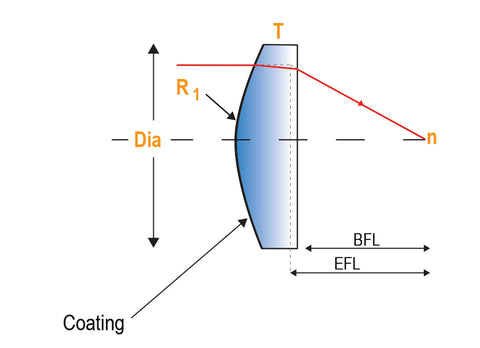Converging or positive lens Curvature on one side of the lens Thicker at its center than at its edges The focal length is defined as positive One focal point  Forming a real or a virtual image

Biconcave lens is the best option when the object and the image are at an infinite conjugate ratio closer to 1:1 with a converging input beam. The output beams will diverge from the virtual image (located on the object side). The focal length is the distance between the virtual image and the lens.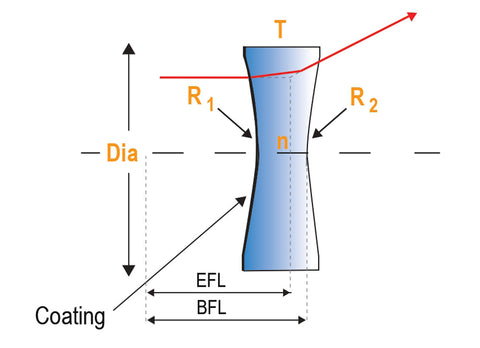Diverging lens Curvature on both sides of the lens Thinner at its center than at its edges The focal length is defined as negative Two focal points Forming a virtual image -  smaller than the object itself

Plano Concave lens is used to expand light or to increase focal lengths or to balance aberrations from other lenses within a system. Spherical aberration and coma are reduced when used at the infinite conjugate ratio with a collimated light incident upon the concave side. Plano concave lens is the best option when the object and the image are at an infinite conjugate ratio greater than 5:1 and less than 1:5. It is recommended to place the curved surface facing the largest object distance to reduce spherical aberration.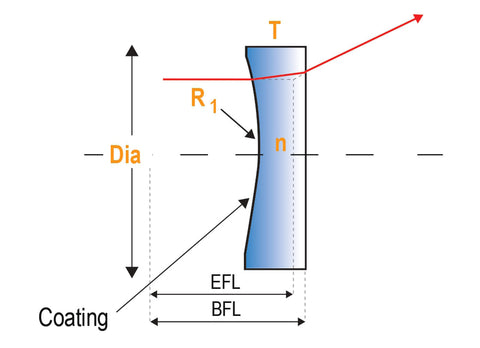Diverging lens Curvature on one side of the lens Thinner at its center than at its edges The focal length is defined as negative One focal point  Forming a virtual image -  smaller than the object itself Increase focal lengths  Balance aberrations from other lenses within a system

Let’s do a comparison between the different lenses

Biconvex Vs Biconcave

Biconvex lenses are focusing light where Biconcave lenses disperse the incident energy.

 Biconvex Biconcave Converging  Curvature on both sides of the lens Thicker at its center than at its edges Positive focal length  Two focal points  Forming a real or a virtual image Used as magnifying or condensing lenses Used as objectives or magnifiers Used in imaging systems such as telescopes, monocular, microscopes, binoculars, cameras, projectors Human eyes  Used in image relays Diverging Curvature on both sides of the lens Thinner at its center than at its edges Negative focal length  Two focal points Forming a virtual image  Used to magnify objects - telescope, peepholes, light projection, and binoculars  Used in eyeglasses - nearsightedness

Biconvex Vs Plano Convex

Both biconvex and plano-convex are positive, converging lenses

 Biconvex Plano Convex Two focal points Finite conjugate - the object being imagined is much closer to the lens Common use - Microscopy One focal point  Infinite conjugate - the object being imaged is far away from the lens Common use -  focusing light from a star Better to deal with spherical aberrations More economic

Biconcave Vs Plano Concave

Both biconcave and plano-concave are negative, diverging lenses

 Biconcave Plano Concave Two focal points  Used in laser beam expanders, optical character readers, viewers, and projection system One focal point  Used to balance aberrations from other lenses within a system

Aspheric lens

The aspheric lens shape is neither spherical nor cylindrical. The radius of curvature changes with distance from the optical axis, unlike a sphere, which has a constant radius.Have a changing radius of curvature  Focus light to a small point Great for imaging - more focused image Used in cameras

Aspheric Vs Spherical

 Aspheric Spherical Changing radius of curvature in certain areas for better light focusing Higher images quality with fewer spherical aberration Smaller, lighter, flatter  Correction of off-axis aberration such as field curvature. Reduction in the number of overall lenses needed to achieve a given result. Fixed radius of curvature Simple design, cheaper to produce Lower images quality when using several spherical lenses. Aberration correction with several spherical lenses reduces the image contrast

### Q&A

What is the difference between Aspheric and Spherical lenses?

Spherical lens has a fixed radius of curvature where Aspheric lens has a changing radius of curvature in certain areas.

Which lens type is cheaper to manufacture?

Aspheric lenses are cheaper to manufacture than Spherical lenses.

Which lens type is converging?

Biconvex and Plano-convex lenses are focussing light where Biconcave and Plano-concave are diverging light.

What is the difference between Biconvex and Plano-convex lenses?

Both Biconvex and Plano-convex are positive, converging lenses. Biconvex lens has two focal points where Plano-convex has one focal point and is cheaper to manufacture.

### Also in Education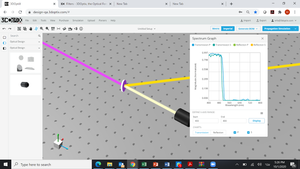October 04, 2020